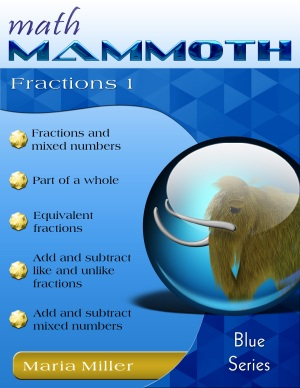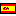^
You are here: HomeVideos → Fraction videos, part 1

# Free fraction videos online, part 1

Enjoy free online math videos on these fraction topics:

(Fraction videos, part 2, are on this page, and cover such topics as simplification, multiplication, and division of fractions.)

The fraction videos on this page match the lessons in my book Math Mammoth Fractions 1 (Blue Series book), or the lessons in chapter 5 of Grade 5-B (Light Blue series). In either book, you will get MANY more practice exercises, word problems, and puzzles than what are shown in the videos, and also some lessons that are not in covered in this set of videos.79 pages (includes answers) PDF download \$3.50 Printed copy \$9.20 Math Mammoth Fractions 1 worktext A self-teaching worktext that focuses on addition and subtraction of fractions & mixed numbers, among other topics. => Learn more!

## Mixed numbers to fractions and vice versaI explain both the visual models and the shortcuts for converting mixed numbers to fractions or fractions to mixed numbers.

To convert a mixed number to a fraction, divide the whole "pies" into pieces (the same kind of pieces as what the fractional part indicates), then figure out how many pieces you have. You can use multiplication, of course.

And to change a fraction into a mixed number, draw enough pies so you can "accommodate" all the fractional parts. The shortcut is, divide. For example, to change 16/5 into a mixed number, divide 16 by 5. That is 3 remainder 1. It means we get 3 whole "pies", and 1 remainder piece, which is a fifth, or 3 1/5.

## Adding and Subtracting Like FractionsI explain how you can teach adding and subtracting like fractions, first using visual models, coloring the crossing out parts, and then advancing into abstract problems. I also include missing addend problems.

## Adding mixed numbers with like fractional partsIn this video we study adding mixed numbers when their fractional parts are like fractions. This is not a difficult concept - all you have to remember is that if the sum of the fractional parts is more than 1, you need to convert that to "whole pies" and whatever is left over.

## Subtract mixed numbers (like fractional parts) - three methodsI show three different methods for subtracting mixed numbers (when we can't just simply subtract the whole-number parts and fractional parts separately):
1. Renaming or borrowing
2. Subtracting in parts
3. Using a negative fraction.

The fractional parts have the same denominator (like fractions), which is the easier situation. It is more complicated if they are unlike fractions - dealt with in another video.

## Equivalent fractions - split the pieces!The visual model for equivalent fractions involves splitting the existing pieces into so many new ones, such as splitting all of them into 4 new pieces. This is shown using the arrow notation with "x4" near the arrows, signifying that the numerator and the denominator get multiplied by 4, because there are now four times as many colored pieces and four times as many pieces in total.

After practicing with visual models, students can go on to abstract problems, still thinking of the arrows in their minds, to help.

## Adding Unlike FractionsThis is a lesson plan (over several lessons) for teaching adding unlike fractions (which I consider to be the most difficult topic in fraction arithmetic).

First, I go through exercises that have a visual model, and the common denominator is given. Then, students should be given exercises without a visual model but the common denominator is still given.

Lastly, we study the rule about finding the common denominator. I also have another video that concentrates solely on that.

## Adding and subtracting mixed numbersFirst, I show two examples with visual models of adding or subtracting mixed numbers. Then we go on to abstract problems, in which we first need to rewrite each problem so that the fractions have a common denominator, and then we can add/subtract. In subtraction, borrowing may be necessary. I also solve one word problem involving a picture frame.

## The least common denominatorThe least common denominator is usually taught together with the topic of adding unlike fractions. Now, I feel that fifth grade students do not yet need to know the exact mechanics of finding the least common denominator, but can simply use any common multiple they find by looking at the multiplication tables of the denominators.

Then, in 6th or 7th grade, students can learn to find the least common multiple of the denominators by making two lists of multiples.

There exists a method for finding the least common multiple of two (or more) numbers that uses prime factoring, but I do not deal with that in this video.

## Comparing fractions - four methodsWhen comparing two fractions, there are three methods that sometimes work:
- If the denominators are the same, compare the amount of pieces
- If the numerators are the same, think of the size of the parts.
- Sometimes you can easily compare to 1/2

If none of those "work", you can always convert the two fractions so they have the same denominator, and then compare.

See also free fraction videos, part 2: simplifying, multiplying, and dividing fractions; fractions to decimals; ratios and fractions, and a fractional part a group of objects.

The videos match the lessons in my book Math Mammoth Fractions 1 (Blue Series book), or the lessons in chapter 5 of Grade 5-B (Light Blue series). In either book, you will get MANY more practice exercises, word problems, and puzzles than what are shown in the videos, and also some lessons that are not in covered in this set of videos.79 pages (includes answers) PDF download \$3.50 Printed copy \$9.20 Math Mammoth Fractions 1 worktext A self-teaching worktext that focuses on addition and subtraction of fractions & mixed numbers, among other topics. => Learn more!

By Maria Miller

WAIT!

Receive my monthly collection of math tips & resources directly in your inbox — and get a FREE Math Mammoth book!You can unsubscribe at any time.

### Math Mammoth Tour

Confused about the different options? Take a virtual email tour around Math Mammoth! You'll receive:

An initial email to download your GIFT of over 400 free worksheets and sample pages from my books. Six other "TOURSTOP" emails that explain the important things and commonly asked questions concerning Math Mammoth curriculum. (Find out the differences between all these different-colored series!)

This way, you'll have time to digest the information over one or two weeks, plus an opportunity to ask me personally about the curriculum.
A monthly collection of math teaching tips & Math Mammoth updates (unsubscribe any time)### "Mini" Math Teaching Course

This is a little "virtual" 2-week course, where you will receive emails on important topics on teaching math, including:

- How to help a student who is behind
- Troubles with word problems
- Teaching multiplication tables
- Why fractions are so difficult
- The value of mistakes
- Should you use timed tests
- And more!

A GIFT of over 400 free worksheets and sample pages from my books right in the very beginning.A monthly collection of math teaching tips & Math Mammoth updates (unsubscribe any time)
Enter your email to receive math teaching tips, resources, Math Mammoth news & sales, humor, and more! I tend to send out these tips about once monthly, near the beginning of the month, but occasionally you may hear from me twice per month (and sometimes less often).• A GIFT of over 400 free worksheets and sample pages from my books.